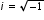# MAFS.912.N-CN.1.1Archived StandardExport Print
Know there is a complex number i such that i² = –1, and every complex number has the form a + bi with a and b real.
General Information
Subject Area: Mathematics
Domain-Subdomain: Number & Quantity: The Complex Number System
Cluster: Level 1: Recall
Cluster: Perform arithmetic operations with complex numbers. (Algebra 2 - Additional Cluster) -

Clusters should not be sorted from Major to Supporting and then taught in that order. To do so would strip the coherence of the mathematical ideas and miss the opportunity to enhance the major work of the grade with the supporting clusters.

Date of Last Rating: 02/14
Status: State Board Approved - Archived

## Related Courses

This benchmark is part of these courses.
1200330: Algebra 2 (Specifically in versions: 2014 - 2015, 2015 - 2022, 2022 and beyond (current))
1200340: Algebra 2 Honors (Specifically in versions: 2014 - 2015, 2015 - 2022, 2022 and beyond (current))
1207310: Liberal Arts Mathematics (Specifically in versions: 2014 - 2015, 2015 - 2022 (course terminated))
1206330: Analytic Geometry (Specifically in versions: 2014 - 2015 (course terminated))
1200335: Algebra 2 for Credit Recovery (Specifically in versions: 2014 - 2015, 2015 - 2019 (course terminated))
7912095: Access Algebra 2 (Specifically in versions: 2016 - 2018, 2018 - 2019, 2019 - 2022, 2022 and beyond (current))

## Related Access Points

Alternate version of this benchmark for students with significant cognitive disabilities.

## Related Resources

Vetted resources educators can use to teach the concepts and skills in this benchmark.

## Lesson Plan

This lesson unit is intended to help you assess how well students are able to:

• Use the properties of exponents, including rational exponents, and manipulate algebraic statements involving radicals.
• Discriminate between equations and identities.

There is also an opportunity to consider the role of the imaginary number, but this is optional.

Type: Lesson Plan

Computations with Complex Numbers:

This resource involves simplifying algebraic expressions that involve complex numbers and various algebraic operations.

## Tutorial

Introduction to i and imaginary numbers:

This video gives an introduction to 'i' and imaginary numbers. From this tutorial, students will learn the rules of imaginary numbers.

Type: Tutorial

## Video/Audio/Animation

MIT BLOSSOMS - Fabulous Fractals and Difference Equations :

This learning video introduces students to the world of Fractal Geometry through the use of difference equations. As a prerequisite to this lesson, students would need two years of high school algebra (comfort with single variable equations) and motivation to learn basic complex arithmetic. Ms. Zager has included a complete introductory tutorial on complex arithmetic with homework assignments downloadable here. Also downloadable are some supplemental challenge problems. Time required to complete the core lesson is approximately one hour, and materials needed include a blackboard/whiteboard as well as space for students to work in small groups. During the in-class portions of this interactive lesson, students will brainstorm on the outcome of the chaos game and practice calculating trajectories of difference equations.

Type: Video/Audio/Animation

## Student Resources

Vetted resources students can use to learn the concepts and skills in this benchmark.

Computations with Complex Numbers:

This resource involves simplifying algebraic expressions that involve complex numbers and various algebraic operations.

## Tutorial

Introduction to i and imaginary numbers:

This video gives an introduction to 'i' and imaginary numbers. From this tutorial, students will learn the rules of imaginary numbers.

Type: Tutorial

## Video/Audio/Animation

MIT BLOSSOMS - Fabulous Fractals and Difference Equations :

This learning video introduces students to the world of Fractal Geometry through the use of difference equations. As a prerequisite to this lesson, students would need two years of high school algebra (comfort with single variable equations) and motivation to learn basic complex arithmetic. Ms. Zager has included a complete introductory tutorial on complex arithmetic with homework assignments downloadable here. Also downloadable are some supplemental challenge problems. Time required to complete the core lesson is approximately one hour, and materials needed include a blackboard/whiteboard as well as space for students to work in small groups. During the in-class portions of this interactive lesson, students will brainstorm on the outcome of the chaos game and practice calculating trajectories of difference equations.

Type: Video/Audio/Animation

## Parent Resources

Vetted resources caregivers can use to help students learn the concepts and skills in this benchmark.